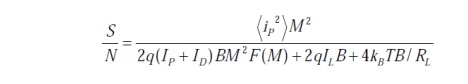Home | | Optical Communication and Networks | Photodetector Noise & S/N

# Photodetector Noise & S/N

Detection of weak optical signal requires that the photodetector and its following amplification circuitry be optimized for a desired signal-to-noise ratio.

Photodetector Noise & S/N:

Detection of weak optical signal requires that the photodetector and its following amplification circuitry be optimized for a desired signal-to-noise ratio.

It is the noise current which determines the minimum optical power level that can be detected. This minimum detectable optical power defines thesensitivity of photodetector. That is the optical power that generates a photocurrent with the amplitude equal to that of the total noise current (S/N=1)## 1. Signal Calculation:

Consider the modulated optical power signal P(t) falls on the photodetector with the form ofWhere s(t) is message electrical signal and m is modulation index. Therefore the primary photocurrent is (for pin photodiode M=1):The root mean square signal current is thenNoise Sources in Photodetecors :

The principal noises associated with photodetectorsare :

1- Quantum (Shot) noise: arises from statistical nature of the production and collection of photo-generated electrons upon optical illumination. It has been shown that the statistics follow a Poisson process.

2- Dark current noise: is the current that continues to flow through the bias circuit in the absence of the light. This is the combination of bulk dark current, which is due to thermally generated e and h in the pn junction, and the surface dark current, due to surface defects, bias voltage an surface area.

In order to calculate the total noise presented in photodetector, we should sum up the root mean square of each noise current by assuming that those are uncorrelated.

Total photodetector noise current=quantum noise current +bulk dark current noise + surface current noise

### Noise calculation :

Quantum noise current (lower limit on the sensitivity):## 2. S/N Calculation:

Having obtained the signal and total noise, the signal-to-noise-ratio can be written as:Since the noise figure F(M) increases with M, there always exists an optimum value of M that maximizes the S/N. For sinusoidally modulated signal with m=1 and F(M) = MxStudy Material, Lecturing Notes, Assignment, Reference, Wiki description explanation, brief detail
Optical Communication and Networking : Sources and Detectors : Photodetector Noise & S/N |